# Test: Electromagnetics - 2

## 20 Questions MCQ Test GATE ECE (Electronics) 2023 Mock Test Series | Test: Electromagnetics - 2

Description
Attempt Test: Electromagnetics - 2 | 20 questions in 60 minutes | Mock test for Electronics and Communication Engineering (ECE) preparation | Free important questions MCQ to study GATE ECE (Electronics) 2023 Mock Test Series for Electronics and Communication Engineering (ECE) Exam | Download free PDF with solutions
QUESTION: 1

### The locus of the resistance 50 Q such that Zo=50Ω for a constructing a smith chart is

Solution:

Radius of the normalized resistance, Centre  =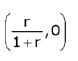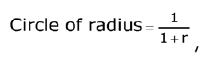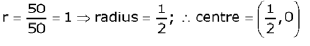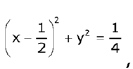Radius = 1/2 and Centre = (1/2, 0)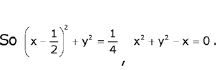QUESTION: 2

### A distortion less transmission line hasZ0 = 50Ω,α = 10mNP/m,u = 0.167C (C = 3x 108m/s'), then R and L of transmission line are

Solution: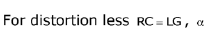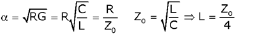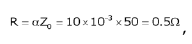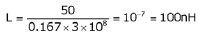QUESTION: 3

### If PSWR is power standing wave ratio, then VSWR and PSWR corresponding to r = 0.5∠450 is

Solution: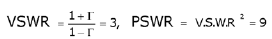QUESTION: 4

Z0 = 600Ω, VSWR = 1.5, the distance at which first maximum occurs is 20cm from the load. If the operating frequency of the transmission line is 300 MHz, then the reflection coefficient is given by

Solution: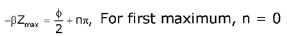For first maximum, n = 0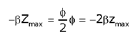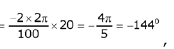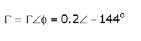QUESTION: 5

For the transmission line shown below, the voltage which appears at the load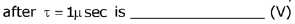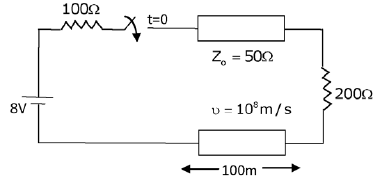Solution: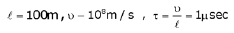All appears at the load. Including this there will some part which is reflected back From the load we should include thisf.QUESTION: 6

A transmission line having a characteristic impedance of 300fl is feeding a dipole antenna. Frequency of operation is 100 MHz and length of dipole antenna is 1.5m.

Q.

Magnitude of reflection coefficient on the line is

Solution: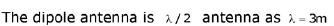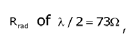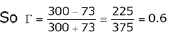QUESTION: 7

A transmission line having a characteristic impedance of 300fl is feeding a dipole antenna. Frequency of operation is 100 MHz and length of dipole antenna is 1.5m.

Q.

If Pout from the transmission line is 100 watts, then the power tramsmitted by the antenna is

Solution: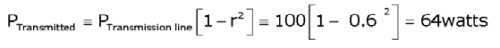QUESTION: 8

Consider the impedance matching series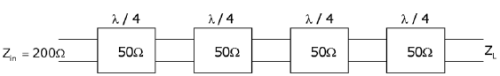Q.

The load impedance ZL is given by

Solution:
QUESTION: 9

Consider the impedance matching seriesQ.

The reflection coefficient at the load end is

Solution: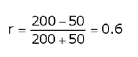QUESTION: 10

A uniform plane wave is incident normally on a dielectric interface with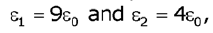the current reflection coefficient, current transmission, current standing wave ration (ISWR) are

Solution: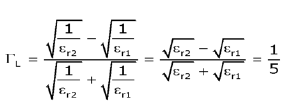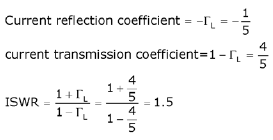QUESTION: 11

Match the following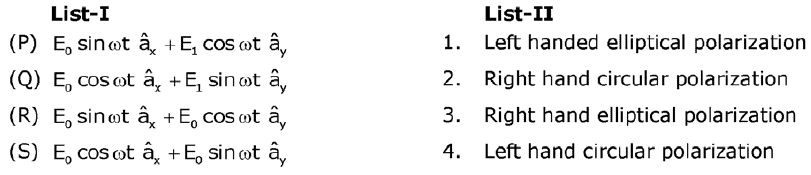Solution: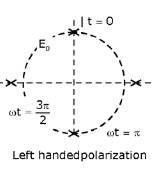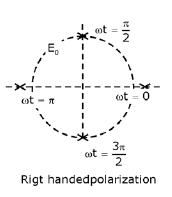If the amplitudes are equal, then it is circularly polarized otherwise elliptical polarization.

QUESTION: 12

In air, a loss less transmission line of length 50 cm with L=10μH/mand C=40 pF/m is operated at 25 MHz. Its electrical path length is

Solution: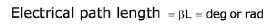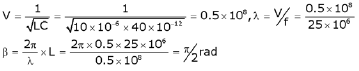QUESTION: 13

A transmission line is lossless and is 25 m long. It is terminated with a load of zL =40+j30Ω at a frequency of 10 MHz. The inductance and capacitance of the line are L - 300nH/m, C = 40pF/m.

Q.

Find β

Solution: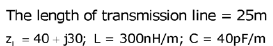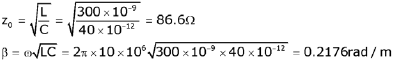QUESTION: 14

A transmission line is lossless and is 25 m long. It is terminated with a load of zL =40+j30Ω at a frequency of 10 MHz. The inductance and capacitance of the line are L - 300nH/m, C = 40pF/m.

Q.

Find the input impedance at the mid-point of the line.

Solution: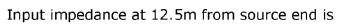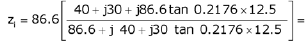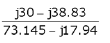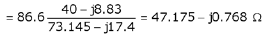QUESTION: 15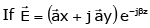then wave is said to be which one of the following,

Solution:
QUESTION: 16

An air filled rectangular wave guide has dimensions a = 2, b = 1 cm, the range of frequencies over which guide will operate in single mode is given by:

Solution:

single mode propagation means only TE|0 mode will propagate.

QUESTION: 17

For a rectangular waveguide of dimensions: a √3 × a (in cm) , if cut-off frequency of TE10 mode is 2GHz. What is the cut-off frequency for TM11 in waveguide?

Solution:
QUESTION: 18

If skin depth at 10 MHz for a conductor is 1/2 π meter then what is the phase velocity for this EM wave?

Solution: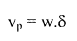QUESTION: 19

The potential distribution is given by V = 10y4 + 2x3. If ε0 is permittivity of free space what is charge density (ρ) at (2, 0)

Solution:
QUESTION: 20

A short vertical grounded antenna is required to radiate at 1 MHz. The effective height of antenna is 302 meter then value of radiation resistance (approximately) is

Solution: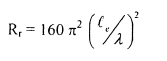Use Code STAYHOME200 and get INR 200 additional OFF Use Coupon Code